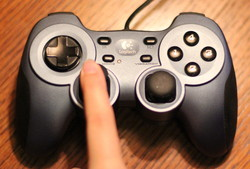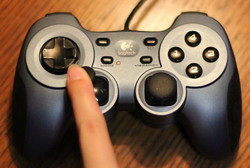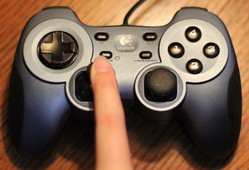# 19. User Control

How do we interact with the user? Get the user to move an object on the screen?

We can do this with the mouse, with the keyboard, or with the game controller. Let’s start with a template with a static ball on the screen:

starting_template.py
 ``` 1 2 3 4 5 6 7 8 9 10 11 12 13 14 15 16 17 18 19 20 21 22 23 24 25 26 27 28 29 30 31 32 33 34 35 36 37 38 39 40 41 42 43 44 45 46 47 48``` ```import arcade SCREEN_WIDTH = 640 SCREEN_HEIGHT = 480 class Ball: def __init__(self, position_x, position_y, radius, color): # Take the parameters of the init function above, # and create instance variables out of them. self.position_x = position_x self.position_y = position_y self.radius = radius self.color = color def draw(self): """ Draw the balls with the instance variables we have. """ arcade.draw_circle_filled(self.position_x, self.position_y, self.radius, self.color) class MyGame(arcade.Window): def __init__(self, width, height, title): # Call the parent class's init function super().__init__(width, height, title) arcade.set_background_color(arcade.color.ASH_GREY) # Create our ball self.ball = Ball(50, 50, 15, arcade.color.AUBURN) def on_draw(self): """ Called whenever we need to draw the window. """ arcade.start_render() self.ball.draw() def main(): window = MyGame(640, 480, "Drawing Example") arcade.run() main() ```

## 19.1. Move with the MouseThe key to managing mouse motion to override the `on_mouse_motion` in the `arcade.Window` class. That method is called every time the mouse moves. The method definition looks like this:

```def on_mouse_motion(self, x, y, dx, dy):
```

The `x` and `y` are the coordinates of the mouse. the `dx` and `dy` represent the change in x and y since the last time the method was called.

Often when controlling a graphical item on the screen with the mouse, we do not want to see the mouse pointer. If you don’t want to see the mouse pointer, in the `__init__` method, call the following method in the parent class:

```self.set_mouse_visible(False)
```

The example below takes our `Ball` class, and moves it around the screen with the mouse.

move_with_mouse.py
 ``` 1 2 3 4 5 6 7 8 9 10 11 12 13 14 15 16 17 18 19 20 21 22 23 24 25 26 27 28 29 30 31 32 33 34 35 36 37 38 39 40 41 42 43 44 45 46 47 48 49 50 51 52 53 54 55 56 57 58``` ```import arcade SCREEN_WIDTH = 640 SCREEN_HEIGHT = 480 class Ball: def __init__(self, position_x, position_y, radius, color): # Take the parameters of the init function above, # and create instance variables out of them. self.position_x = position_x self.position_y = position_y self.radius = radius self.color = color def draw(self): """ Draw the balls with the instance variables we have. """ arcade.draw_circle_filled(self.position_x, self.position_y, self.radius, self.color) class MyGame(arcade.Window): def __init__(self, width, height, title): # Call the parent class's init function super().__init__(width, height, title) # Make the mouse disappear when it is over the window. # So we just see our object, not the pointer. self.set_mouse_visible(False) arcade.set_background_color(arcade.color.ASH_GREY) # Create our ball self.ball = Ball(50, 50, 15, arcade.color.AUBURN) def on_draw(self): """ Called whenever we need to draw the window. """ arcade.start_render() self.ball.draw() def on_mouse_motion(self, x, y, dx, dy): """ Called to update our objects. Happens approximately 60 times per second.""" self.ball.position_x = x self.ball.position_y = y def main(): window = MyGame(640, 480, "Drawing Example") arcade.run() main() ```

## 19.2. Mouse Clicks

You can also process mouse clicks by defining an `on_mouse_press` method:

```def on_mouse_press(self, x, y, button, modifiers):
""" Called when the user presses a mouse button. """

print("Left mouse button pressed at", x, y)
print("Right mouse button pressed at", x, y)
```

## 19.3. Move with the KeyboardMoving with the game controller is similar to our bouncing ball example. There are just two differences:

• We control the `change_x` and `change_y` with the keyboard

• When we hit the edge of the screen we stop, rather than bounce.

To detect when a key is hit, we override the `on_key_press` method. We might think of hitting a key as one event. But it is actually two. When the key is pressed, we start moving. When the key is released we stop moving. That makes for two events. Releasing a key is controlled by `on_key_release`.

These methods have a `key` variable as a parameter that can be compared with an `if` statement to the values in the arcade.key library.

```def on_key_press(self, key, modifiers):
print("Left key hit")
print("The 'a' key was hit")
```

We can use this in a program to move a ball around the screen. See the highlighted lines in the program below:

move_with_keyboard_simple.py
 ``` 1 2 3 4 5 6 7 8 9 10 11 12 13 14 15 16 17 18 19 20 21 22 23 24 25 26 27 28 29 30 31 32 33 34 35 36 37 38 39 40 41 42 43 44 45 46 47 48 49 50 51 52 53 54 55 56 57 58 59 60 61 62 63 64 65 66 67 68 69 70 71 72 73 74 75 76 77 78 79 80 81``` ```import arcade SCREEN_WIDTH = 640 SCREEN_HEIGHT = 480 MOVEMENT_SPEED = 3 class Ball: def __init__(self, position_x, position_y, change_x, change_y, radius, color): # Take the parameters of the init function above, # and create instance variables out of them. self.position_x = position_x self.position_y = position_y self.change_x = change_x self.change_y = change_y self.radius = radius self.color = color def draw(self): """ Draw the balls with the instance variables we have. """ arcade.draw_circle_filled(self.position_x, self.position_y, self.radius, self.color) def update(self): # Move the ball self.position_y += self.change_y self.position_x += self.change_x class MyGame(arcade.Window): def __init__(self, width, height, title): # Call the parent class's init function super().__init__(width, height, title) # Make the mouse disappear when it is over the window. # So we just see our object, not the pointer. self.set_mouse_visible(False) arcade.set_background_color(arcade.color.ASH_GREY) # Create our ball self.ball = Ball(50, 50, 0, 0, 15, arcade.color.AUBURN) def on_draw(self): """ Called whenever we need to draw the window. """ arcade.start_render() self.ball.draw() def update(self, delta_time): self.ball.update() def on_key_press(self, key, modifiers): """ Called whenever the user presses a key. """ if key == arcade.key.LEFT: self.ball.change_x = -MOVEMENT_SPEED elif key == arcade.key.RIGHT: self.ball.change_x = MOVEMENT_SPEED elif key == arcade.key.UP: self.ball.change_y = MOVEMENT_SPEED elif key == arcade.key.DOWN: self.ball.change_y = -MOVEMENT_SPEED def on_key_release(self, key, modifiers): """ Called whenever a user releases a key. """ if key == arcade.key.LEFT or key == arcade.key.RIGHT: self.ball.change_x = 0 elif key == arcade.key.UP or key == arcade.key.DOWN: self.ball.change_y = 0 def main(): window = MyGame(640, 480, "Drawing Example") arcade.run() main() ```

### 19.3.1. Keep From Moving Off ScreenUnfortunately in the prior program, there is nothing that keeps the player from moving off-screen. If we want to stop the player from moving off-screen we need some additional code.

We detect the edge by comparing `position_x` with the left and right side of the screen For example:

```if self.position_x < 0:
```

But this isn’t perfect. Because the position specifies the center of the ball, by the time the x coordinate is 0 we are already have off the screen. It is better to compare it to the ball’s radius:

```if self.position_x < self.radius:
```

What do we do once it hits the edge? Just set the value back to the edge:

```# See if the ball hit the edge of the screen. If so, change direction
```

Here’s a full example:

move_with_keyboard_edge_detect.py
 ``` 1 2 3 4 5 6 7 8 9 10 11 12 13 14 15 16 17 18 19 20 21 22 23 24 25 26 27 28 29 30 31 32 33 34 35 36 37 38 39 40 41 42 43 44 45 46 47 48 49 50 51 52 53 54 55 56 57 58 59 60 61 62 63 64 65 66 67 68 69 70 71 72 73 74 75 76 77 78 79 80 81 82 83 84 85 86 87 88 89 90 91 92 93 94``` ```import arcade SCREEN_WIDTH = 640 SCREEN_HEIGHT = 480 MOVEMENT_SPEED = 3 class Ball: def __init__(self, position_x, position_y, change_x, change_y, radius, color): # Take the parameters of the init function above, # and create instance variables out of them. self.position_x = position_x self.position_y = position_y self.change_x = change_x self.change_y = change_y self.radius = radius self.color = color def draw(self): """ Draw the balls with the instance variables we have. """ arcade.draw_circle_filled(self.position_x, self.position_y, self.radius, self. color) def update(self): # Move the ball self.position_y += self.change_y self.position_x += self.change_x # See if the ball hit the edge of the screen. If so, change direction if self.position_x < self.radius: self.position_x = self.radius if self.position_x > SCREEN_WIDTH - self.radius: self.position_x = SCREEN_WIDTH - self.radius if self.position_y < self.radius: self.position_y = self.radius if self.position_y > SCREEN_HEIGHT - self.radius: self.position_y = SCREEN_HEIGHT - self.radius class MyGame(arcade.Window): def __init__(self, width, height, title): # Call the parent class's init function super().__init__(width, height, title) # Make the mouse disappear when it is over the window. # So we just see our object, not the pointer. self.set_mouse_visible(False) arcade.set_background_color(arcade.color.ASH_GREY) # Create our ball self.ball = Ball(50, 50, 0, 0, 15, arcade.color.AUBURN) def on_draw(self): """ Called whenever we need to draw the window. """ arcade.start_render() self.ball.draw() def update(self, delta_time): self.ball.update() def on_key_press(self, key, modifiers): """ Called whenever the user presses a key. """ if key == arcade.key.LEFT: self.ball.change_x = -MOVEMENT_SPEED elif key == arcade.key.RIGHT: self.ball.change_x = MOVEMENT_SPEED elif key == arcade.key.UP: self.ball.change_y = MOVEMENT_SPEED elif key == arcade.key.DOWN: self.ball.change_y = -MOVEMENT_SPEED def on_key_release(self, key, modifiers): """ Called whenever a user releases a key. """ if key == arcade.key.LEFT or key == arcade.key.RIGHT: self.ball.change_x = 0 elif key == arcade.key.UP or key == arcade.key.DOWN: self.ball.change_y = 0 def main(): window = MyGame(640, 480, "Drawing Example") arcade.run() main() ```

## 19.4. Moving with the Game ControllerWorking with game controllers is a bit more complex. A computer might not have any game controllers, or it might have five controllers plugged in.

We can get a list of all game pads that are plugged in with the `arcade.get_joysticks()` function. This will either return a list, or it will return nothing at all if there are no game pads.

Below is a block of code that can be put in an `__init__` method for your application that will create an instance variable to represent a game pad if one exists.

```joysticks = arcade.get_joysticks()
if joysticks:
self.joystick = joysticks
self.joystick.open()
else:
print("There are no joysticks.")
self.joystick = None
```

## 19.5. Joystick Values

After this, you can get the position of the game controller joystick by calling `self.joystick.x` and `self.joystick.y`.

Try this, combined with the initialization code from above:

move_with_game_controller_print.py
 ``` 1 2 3 4 5 6 7 8 9 10 11 12 13 14 15 16 17 18 19 20 21 22 23 24 25 26 27 28 29 30 31 32 33 34 35 36 37 38 39 40 41 42 43 44 45 46 47 48 49 50 51 52 53 54 55 56 57 58 59 60 61 62 63 64 65 66 67 68 69 70 71 72 73 74 75 76 77 78 79 80 81 82 83 84 85 86 87 88 89 90 91 92 93``` ```import arcade SCREEN_WIDTH = 640 SCREEN_HEIGHT = 480 MOVEMENT_SPEED = 5 DEAD_ZONE = 0.02 class Ball: def __init__(self, position_x, position_y, change_x, change_y, radius, color): # Take the parameters of the init function above, # and create instance variables out of them. self.position_x = position_x self.position_y = position_y self.change_x = change_x self.change_y = change_y self.radius = radius self.color = color def draw(self): """ Draw the balls with the instance variables we have. """ arcade.draw_circle_filled(self.position_x, self.position_y, self.radius, self.color) def update(self): # Move the ball self.position_y += self.change_y self.position_x += self.change_x # See if the ball hit the edge of the screen. If so, change direction if self.position_x < self.radius: self.position_x = self.radius if self.position_x > SCREEN_WIDTH - self.radius: self.position_x = SCREEN_WIDTH - self.radius if self.position_y < self.radius: self.position_y = self.radius if self.position_y > SCREEN_HEIGHT - self.radius: self.position_y = SCREEN_HEIGHT - self.radius class MyGame(arcade.Window): def __init__(self, width, height, title): # Call the parent class's init function super().__init__(width, height, title) # Make the mouse disappear when it is over the window. # So we just see our object, not the pointer. self.set_mouse_visible(False) arcade.set_background_color(arcade.color.ASH_GREY) # Create our ball self.ball = Ball(50, 50, 0, 0, 15, arcade.color.AUBURN) # Get a list of all the game controllers that are plugged in joysticks = arcade.get_joysticks() # If we have a game controller plugged in, grab it and # make an instance variable out of it. if joysticks: self.joystick = joysticks self.joystick.open() else: print("There are no joysticks.") self.joystick = None def on_draw(self): """ Called whenever we need to draw the window. """ arcade.start_render() self.ball.draw() def update(self, delta_time): # Update the position according to the game controller if self.joystick: print(self.joystick.x, self.joystick.y) def main(): window = MyGame(640, 480, "Drawing Example") arcade.run() main() ```

Run the program and see the values it prints out for your game controller as you move the joystick on it around.

• The values will be between -1 and +1, with 0 being a centered joystick.

• The x-axis numbers will be negative if the stick goes left, positive for right.

• The y-axis numbers will be opposite of what you might expect. Negative for up, positive for down.Centered (0, 0)Down (0, 1)Down/Left (-1, 1)Down/Right (1, 1)Up (0, -1)Up/Left (-1, -1)Up/Right (1, -1)

We can move the ball by adding the following code to the `update`:

```def update(self, delta_time):

# Update the position according to the game controller
if self.joystick:
print(self.joystick.x, self.joystick.y)

self.ball.change_x = self.joystick.x
self.ball.change_y = -self.joystick.y
```

Notice the `-` we put in front of setting the y vector. If we don’t do this, the ball will move opposite of what we expect when going up/down. This is because the joystick has y values mapped opposite of how we’d normally expect. There’s a long story to that, which I will not bore you with now.

But with this code our ball moves so slow. How do we speed it up? We can make it run five times faster by multiplying by five if we want.

```def update(self, delta_time):

# Update the position according to the game controller
if self.joystick:
print(self.joystick.x, self.joystick.y)

self.ball.change_x = self.joystick.x * 5
self.ball.change_y = -self.joystick.y * 5
```

Or better yet, define a constant variable at the top of your program and use that. In our final example below, we’ll do just that.

 ``` 1 2 3 4 5 6 7 8 9 10 11 12 13 14 15 16 17 18 19 20 21 22 23 24 25 26 27 28 29 30 31 32 33 34 35 36 37 38 39 40 41 42 43 44 45 46 47 48 49 50 51 52 53 54 55 56 57 58 59 60 61 62 63 64 65 66 67 68 69 70 71 72 73 74 75 76 77 78 79 80 81 82 83 84 85 86 87 88 89 90 91 92 93 94 95 96 97 98 99 100 101 102 103 104 105 106``` ```import arcade SCREEN_WIDTH = 640 SCREEN_HEIGHT = 480 MOVEMENT_SPEED = 5 DEAD_ZONE = 0.02 class Ball: def __init__(self, position_x, position_y, change_x, change_y, radius, color): # Take the parameters of the init function above, # and create instance variables out of them. self.position_x = position_x self.position_y = position_y self.change_x = change_x self.change_y = change_y self.radius = radius self.color = color def draw(self): """ Draw the balls with the instance variables we have. """ arcade.draw_circle_filled(self.position_x, self.position_y, self.radius, self.color) def update(self): # Move the ball self.position_y += self.change_y self.position_x += self.change_x # See if the ball hit the edge of the screen. If so, change direction if self.position_x < self.radius: self.position_x = self.radius if self.position_x > SCREEN_WIDTH - self.radius: self.position_x = SCREEN_WIDTH - self.radius if self.position_y < self.radius: self.position_y = self.radius if self.position_y > SCREEN_HEIGHT - self.radius: self.position_y = SCREEN_HEIGHT - self.radius class MyGame(arcade.Window): def __init__(self, width, height, title): # Call the parent class's init function super().__init__(width, height, title) # Make the mouse disappear when it is over the window. # So we just see our object, not the pointer. self.set_mouse_visible(False) arcade.set_background_color(arcade.color.ASH_GREY) # Create our ball self.ball = Ball(50, 50, 0, 0, 15, arcade.color.AUBURN) # Get a list of all the game controllers that are plugged in joysticks = arcade.get_joysticks() # If we have a game controller plugged in, grab it and # make an instance variable out of it. if joysticks: self.joystick = joysticks self.joystick.open() else: print("There are no joysticks.") self.joystick = None def on_draw(self): """ Called whenever we need to draw the window. """ arcade.start_render() self.ball.draw() def update(self, delta_time): # Update the position according to the game controller if self.joystick: # Set a "dead zone" to prevent drive from a centered joystick if abs(self.joystick.x) < DEAD_ZONE: self.ball.change_x = 0 else: self.ball.change_x = self.joystick.x * MOVEMENT_SPEED # Set a "dead zone" to prevent drive from a centered joystick if abs(self.joystick.y) < DEAD_ZONE: self.ball.change_y = 0 else: self.ball.change_y = -self.joystick.y * MOVEMENT_SPEED self.ball.update() def main(): window = MyGame(640, 480, "Drawing Example") arcade.run() main() ```